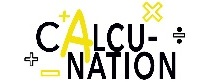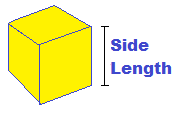Math isn't easy for everyone,
CalcuNation is.

# Cube Surface Area Calculator

## Find the surface area of a cube with this Cube Surface Area Calculator.Side Length:
Fill in the length of one of the cube sides to find the cube surface area.

### How do you find the surface area of a cube?

To find the surface area of a cube, use the equation:
Cube Surface Area = 6 x Side Length2

Example: The surface area of a cube with a side length of 3 inches is:
Cube Surface Area = 6 x 32

Calculated out this gives a surface area of 54 Square Inches.